Updated on 2021-12-15: Include ordered constraint.

This post discusses some latent analysis techniques and runs a Bayesian analysis for example data where the outcome is continuous, also known as latent profile analysis (LPA). My aim will be to clearly visualize the analysis so that it can easily be adjusted to different contexts.

In essence, latent analyses about finding hidden groups in data (Oberski, 2016). Specifically, they are called mixture models because the underlying distributions are mixed together.

For example, suppose we had data about dog weights and did not register the breed. If we assume that the sample only consists of Australian Sheperds and American Terriers, that American Terriers are larger on average, and that both groups are normally distributed, then we can take the observed data and estimate the latent distributions. For example, we can generate some data:

``````begin
using Bijectors: OrderedBijector, ordered, inv
using CairoMakie
using DataFrames
using StableRNGs: StableRNG
using Statistics: mean, median, std
using Turing
end``````
``d1 = Normal(22, 2.4); # Australian Shepherd.``
``d2 = Normal(28, 2.8); # American Terrier.``
``````combined = let
rng = StableRNG(1)
[i % 2 == 1 ? rand(rng, d1) : rand(rng, d2) for i in 1:200]
end;``````

and visualize it: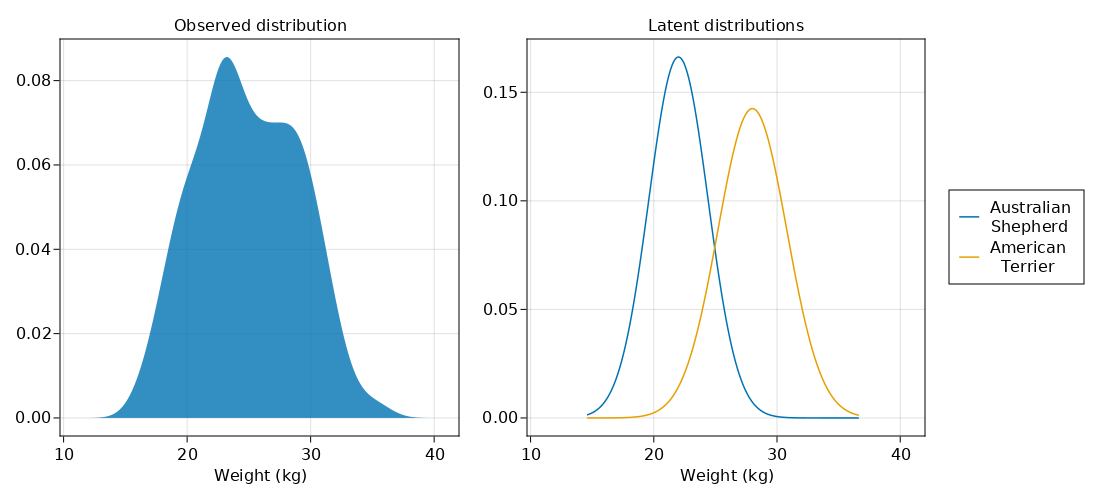Next to LPA, this problem is also known as gaussian (finite) mixture modelling. When the observed variables are discrete, the appropriate model is known as latent class analysis (LCA) or binomial (finite) mixture model. LCA is also known as latent Dirichlet allocation in the machine learning literature.

As a sidenote, Latent Dirichlet allocation is one of the older methods used for natural language processing tasks (Blei et al., 2003). By old, here, I mean that it is one of the methods used before the advent of deep learning around 2013. A typical natural language processing tasks would be to classify documents. In this setting, LDA can be used to interpret text by finding words with a similar "topic". For example, the topic can be "education" with words such as "school", "students", "schools" and "teachers" (Blei et al., 2003). This example also shows one of the main differences between LDA usage in machine learning and LCA usage in science. Both are essentially the same models, but where social science often sticks to a few latent classes to manually interpret, machine learning happily runs the model for 100 latent classes (Blei et al., 2003; p. 1007).

## First attempt

Enough background, let's fit a latent profile model via Turing. Based on the Turing tutorial and a great Discourse post, we can fit a nice model which runs in less than a minute:

``````@model function exchangeable_mixture(k::Int, Y)
w ~ Dirichlet(k, 1)

μ ~ filldist(Normal(25, 4), 2)

n = length(Y)
Y ~ filldist(MixtureModel(Normal, μ, w), n)
end;``````
``exchangeable_model = exchangeable_mixture(2, combined);``
``n_samples = 1_000;``

Just to be sure, let's sample from the prior to see how things look:

``exchangeable_prior = sample(exchangeable_model, Prior(), n_samples);``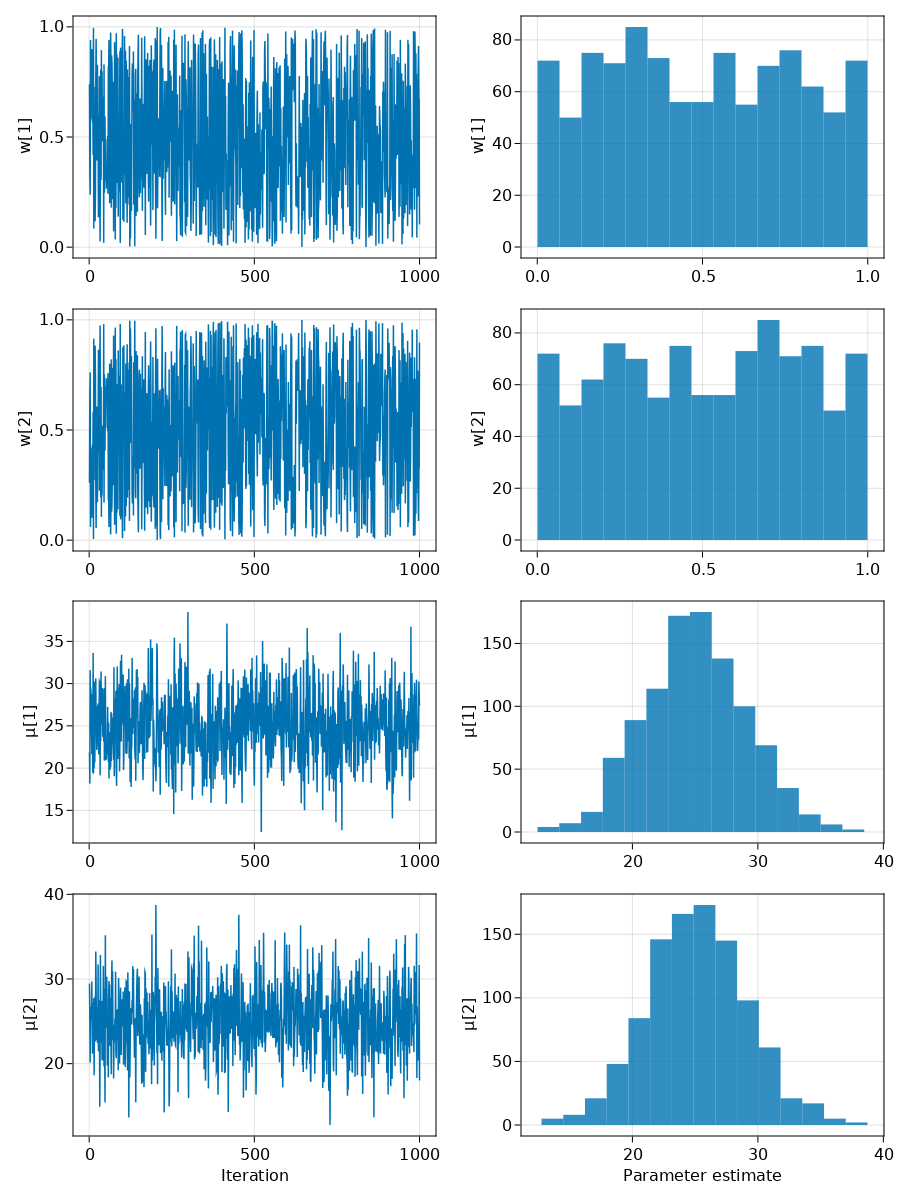This looks as expected. When looking at the observed distribution in the first figure in this blog, it is not unreasonable to set the means for the `μ` priors around the middle of the data. With that, the sampler has a nice place to start and should be able to estimate the parameters reasonably quickly.

The `w` specifies the weights of the latent classes. In the `combined` data, I've drawn half of the samples from `d1` and half of the samples from `d2`, so the weights should be 0.5 and 0.5. This Dirichlet prior is a, so called, unit simplex. To me it just looks like an uniform prior, but I guess there is a good reason for the unit simplex term. This prior is reasonable because it doesn't tell the sampler much about the location of the weights except that they are between 0 and 1.

So, the prior look good. It's time to obtain the posterior. To do this, we need to use HMC and not NUTS. Normally, NUTS is the best sampler to use, but for latent models NUTS is often having problems. Online, people seem to suggest that this is due to the multimodality, that is, because there are two solutions to this problem:

1. μ1 estimates the mean of Sheperds and μ2 estimates the mean of Terriers OR

2. μ2 estimates the mean of Teriers and μ2 estimates the mean of Shepers.

This is called the identifiability problem (Casella & Berger, 2002), the label switching problem (Obserki, 2016) or labeling degeneracies (Betancourt, 2017).

So, let's sample 3 chains in parallel with `HMC`:

``````exchangable_posterior = let
rng = StableRNG(1)
sampler = HMC(0.2, 10)
sample(rng, exchangeable_model, sampler, MCMCThreads(), n_samples, 3)
end;``````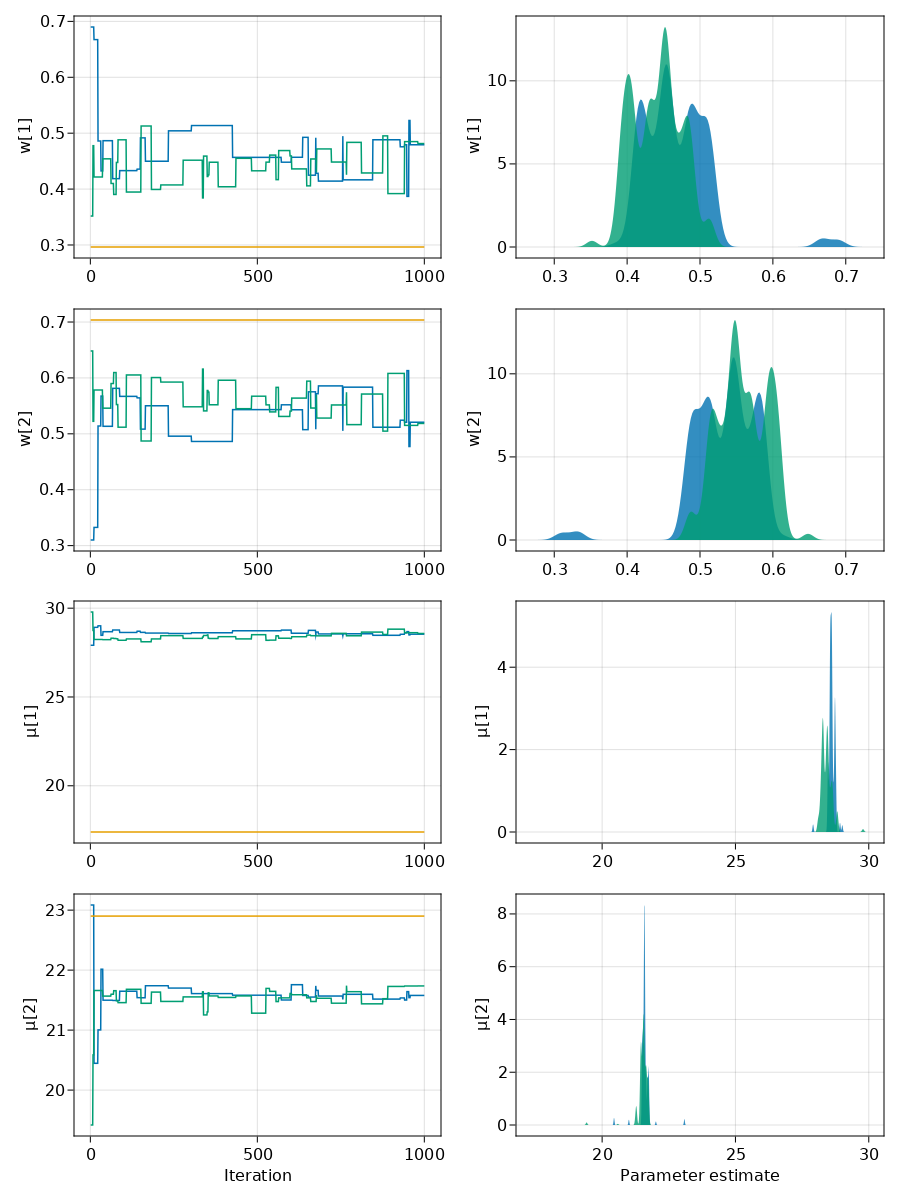Hmm. That didn't work. When the one or more chains don't move at all (a horizontal line in the left plot) for mixture models, then try reducing the leapfrog step size (the first argument to `HMC`).

``````exchangable_posterior_smaller_stepsize = let
rng = StableRNG(1)
sampler = HMC(0.01, 10)
sample(rng, exchangeable_model, sampler, MCMCThreads(), n_samples, 4)
end;``````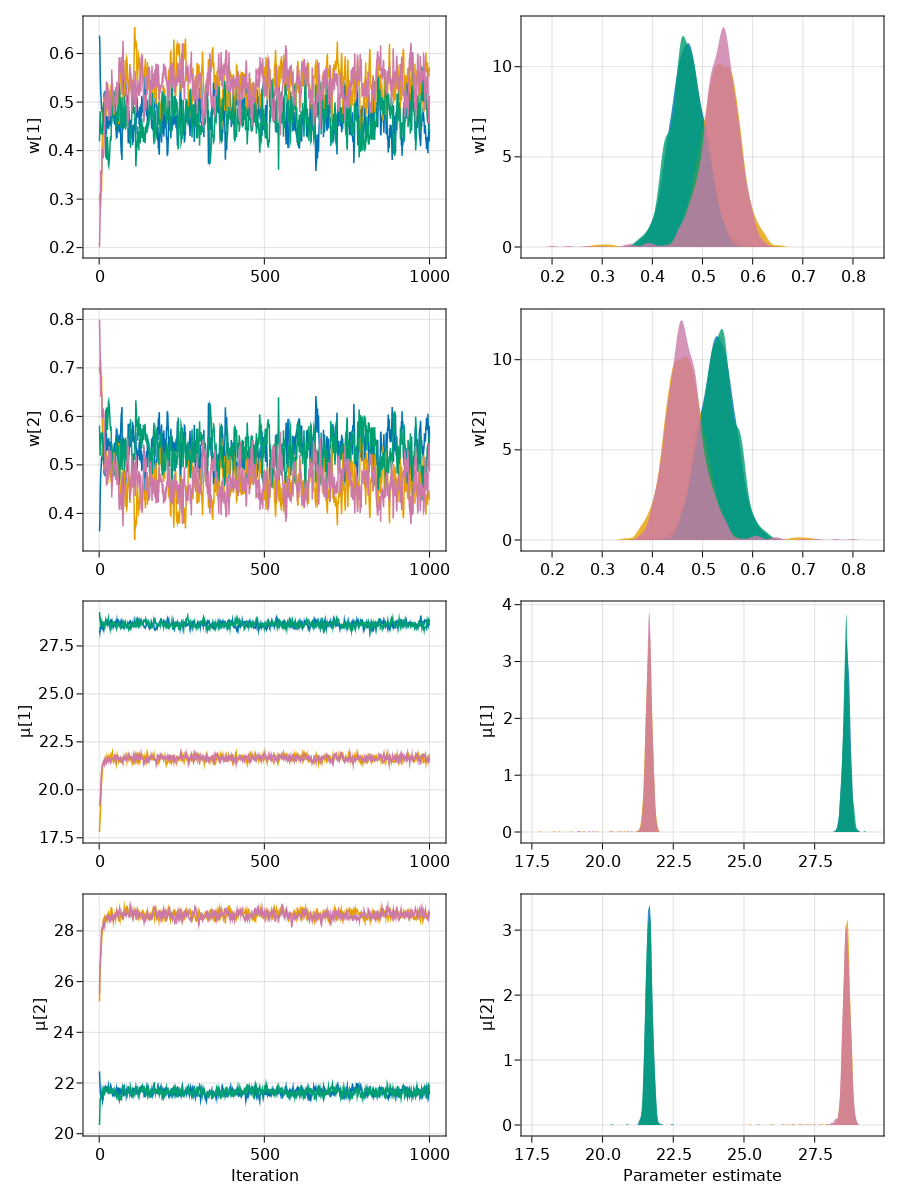That looks much better. However, now we're dealing with the label switching problem. Normally, to get the parameter estimate, we could just take the mean over all the chains. In this case, we couldn't do that and instead should take the mean over only one chain? That would work, but isn't ideal either.

## Fixing the label switching

Betancourt (2017) suggests using an ordered prior for μ. Via `Bijectors.jl.OrderedBijector` this should be possible in `Turing.jl` too. Unfortunately, I wasn't able to figure it out. (It appears that the Stan model is transforming things to the log scale and that works well together with the ordered prior. I'm too lazy to convert things to the log scale and back again, so that's why I'm not doing that.)

As a workaround, I came up with the idea to enforce the ordering in another way, namely to cutoff the range of possible values via two non-overlapping uniform distributions. This can be thought of as drawing a line through the middle of the two means which will lock both parameters in their own region.

``````@model function mm(k::Int, Y)
w ~ Dirichlet(k, 1)

μ1 = Uniform(10, 25)
μ2 = Uniform(25, 40)
μ ~ arraydist([μ1, μ2])

n = length(Y)
Y ~ filldist(MixtureModel(Normal, μ, w), n)
end;``````
``mixture_model = mm(2, combined);``
``mixture_model_prior = sample(mixture_model, Prior(), n_samples);``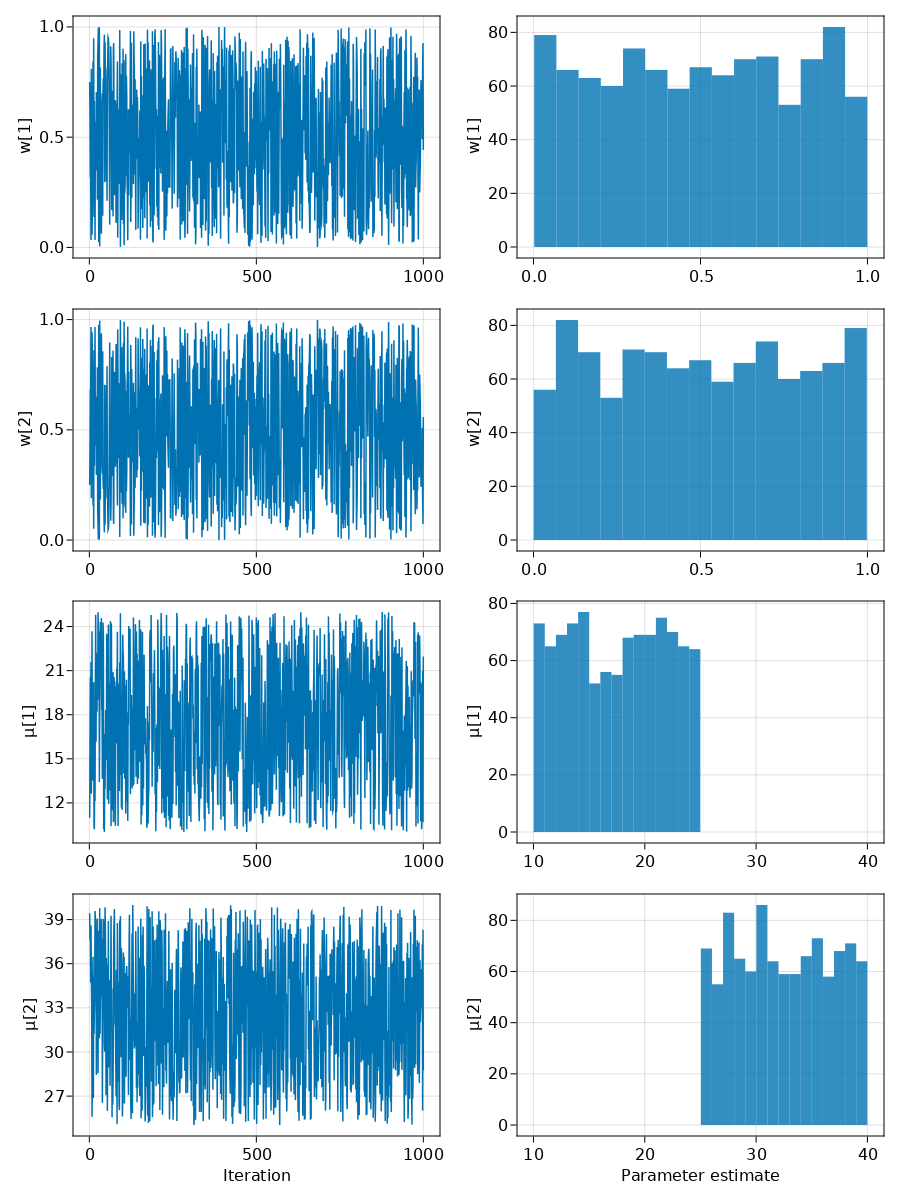After a bit of fiddling and increasing the number of leapfrog steps to use (the second argument to `HMC`), this shows chains with nice convergence and mixing:

``````mixture_posterior = let
rng = StableRNG(1)
sampler = HMC(0.01, 20)
sample(rng, mixture_model, sampler, MCMCThreads(), n_samples, 3)
end;``````